# Lions Weekly: September 22, 2021# A Note From Mr. Sandefur

Lion Family,

For those who follow the news, you may be aware that recently students throughout the nation have been bombarded with cultural trends and influences that do not align with Biblical values and that are leading to real-life consequences. I was blessed to have several conversations with students this week as we discussed the importance of not following the trends of this world, but instead relying heavily upon the truths of the gospel. Our children face so many obstacles that the generation prior never had to face. Therefore, our goal at TKA is to come alongside our families and instill Biblical principles that will help students stand firm in the face of adversity and temptation.

If you were unable to travel to Lebanon, TN last Friday for our region football game against Friendship Christian, you missed an incredible evening! We are very proud of our Lions and all of those who made the long ride to see us defeat FC for the first time in school history. While our boys have had success on the field lately, the rest of our fall student-athletes have been shining as well. Volleyball is competing hard, and several of our golfers qualified for the region competition. I am deeply thankful for the success of our students in the classroom, on the fields of competition, and within our community.

Go Lions!

In Christ Alone,

Jeremy Sandefur

President

 table div table+table+table+table div table{width:100%;padding:0}table div table+table+table+table div table img{width:96.23%;padding:0;float:none}table div table+table+table+table div table td{width:100%;padding:0 1.88% 18px}/* styles */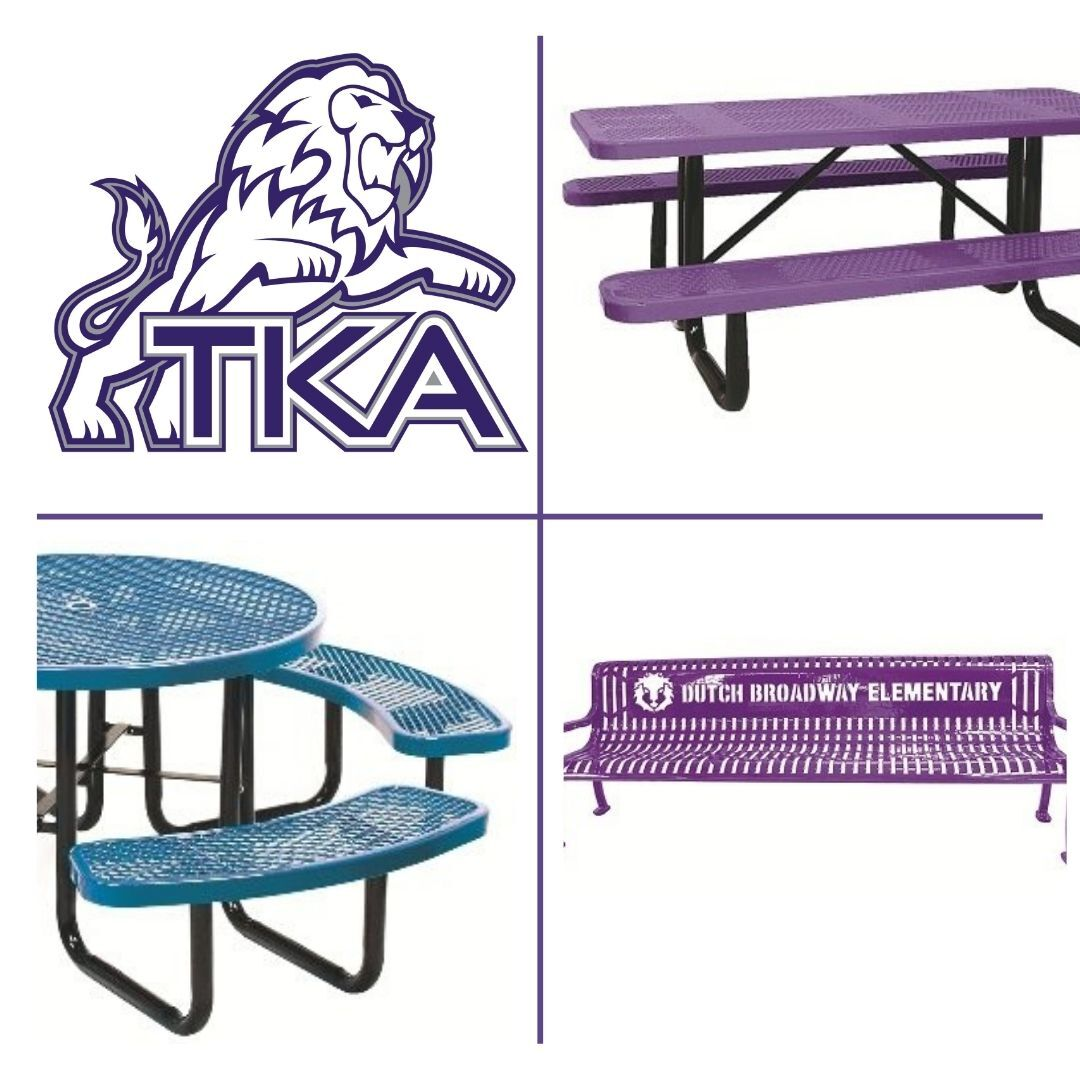# Dr. Carroll Reece Reagan Pavilion

The Dr. Carroll Reece Reagan Pavilion is nearing completion (aiming for the next few weeks), and we are in need of a few donations to furnish the space for our students. Upon completion, the Reagan Pavilion will be a greatly used outdoor spot on campus for covered meals, class meetings, fellowships, and more. If you, or your company, are interested in meeting one of these needs for our students you may either use the below link (list Pavilion table in the note) or contact the school office at (865) 573-8321. The following choices have been made based on durability and function. These will greatly add to this exciting spot on campus! All sponsors are greatly appreciated and will be listed within the Pavilion.

10ft purple tables (6 tables, 1 already sponsored leaving a need for 5)
\$1,300

46 inch round purple tables
\$850

Personalized Benches

6ft benches (2 benches) \$1,200

We are over halfway finished with our goal! Thank you to all who have given so graciously thus far!

 /* styles */ As the Dr. Carroll Reece Reagan Pavilion is nearing completion, faculty, staff and students took time to cover the walls with scripture. We are thankful for all God is doing on our campus at TKA!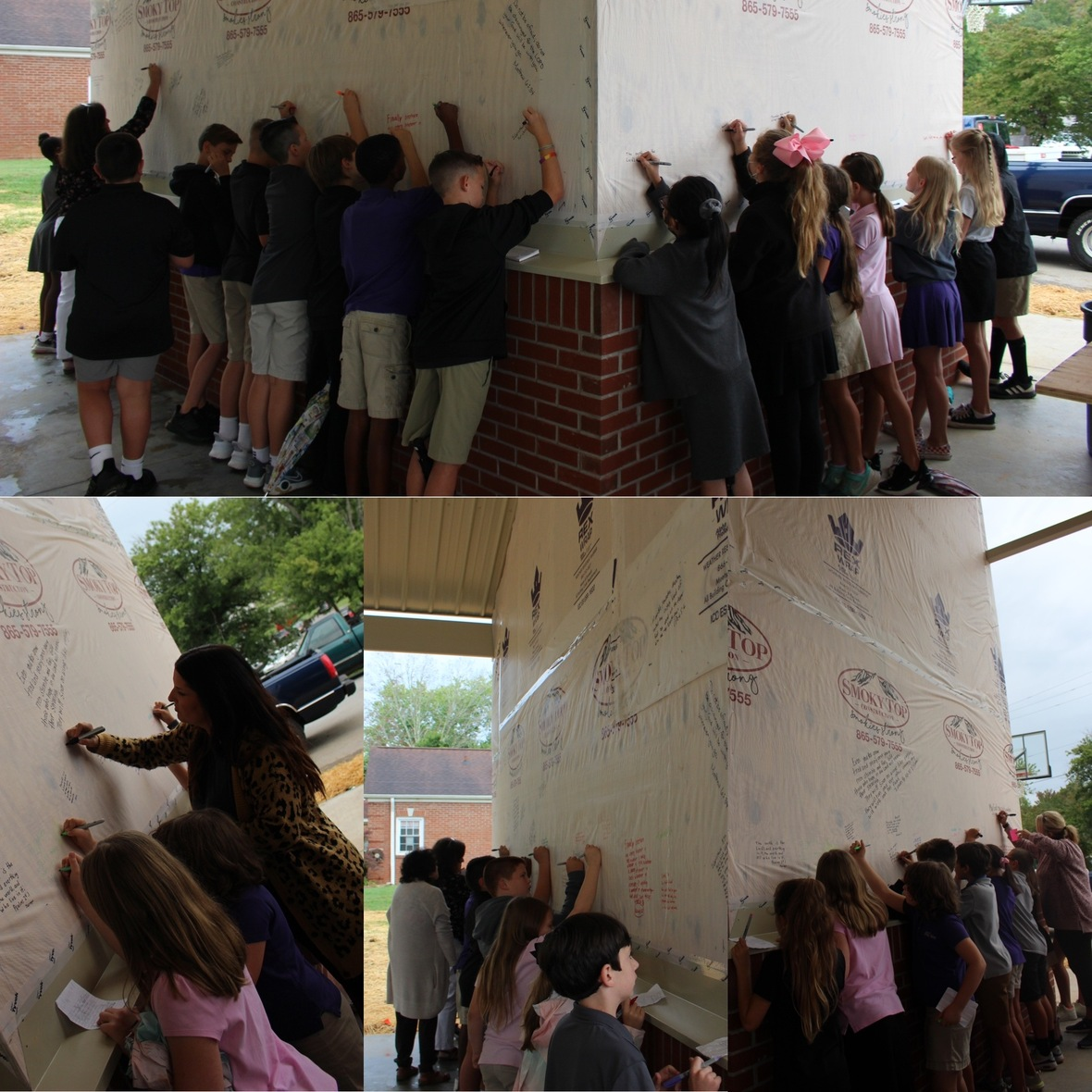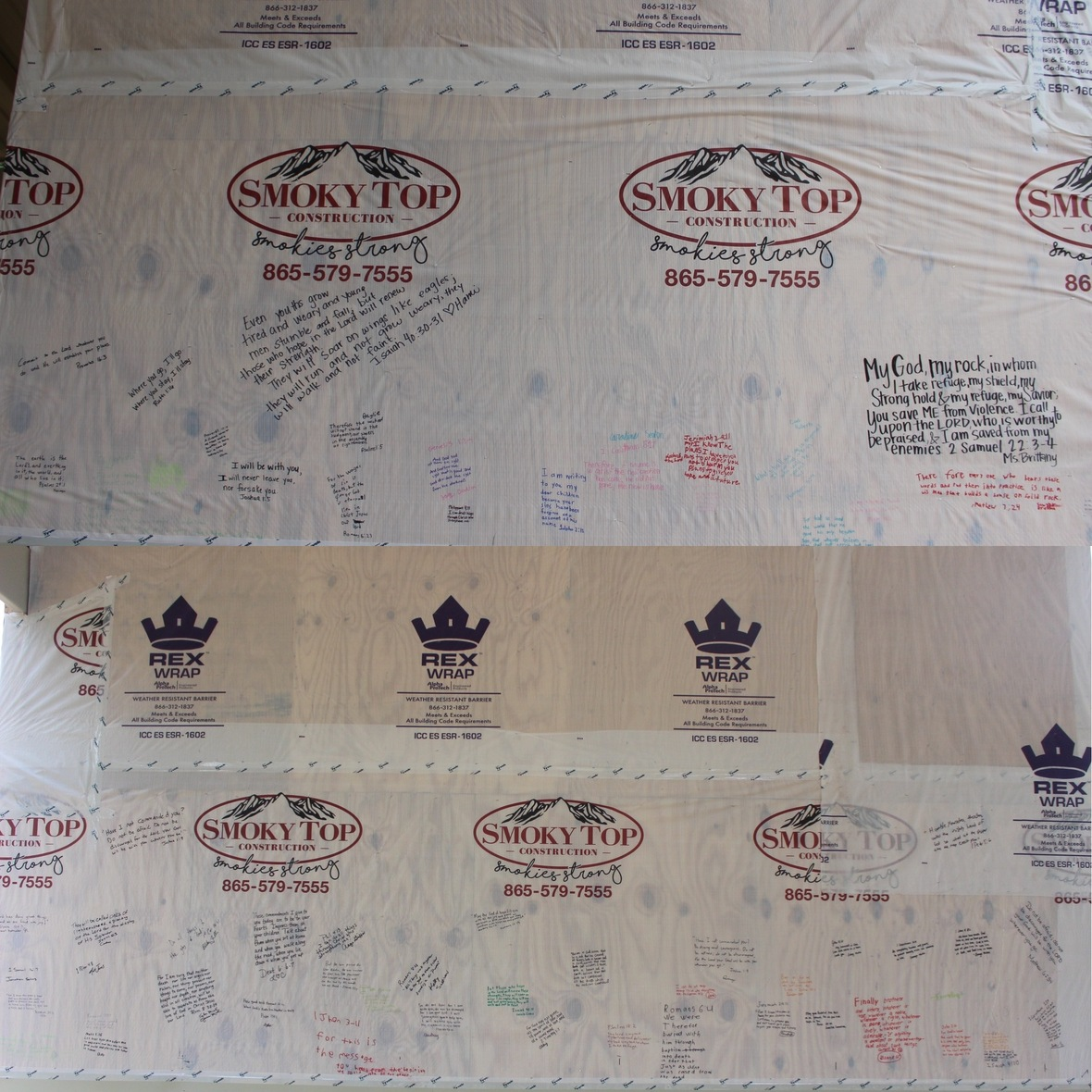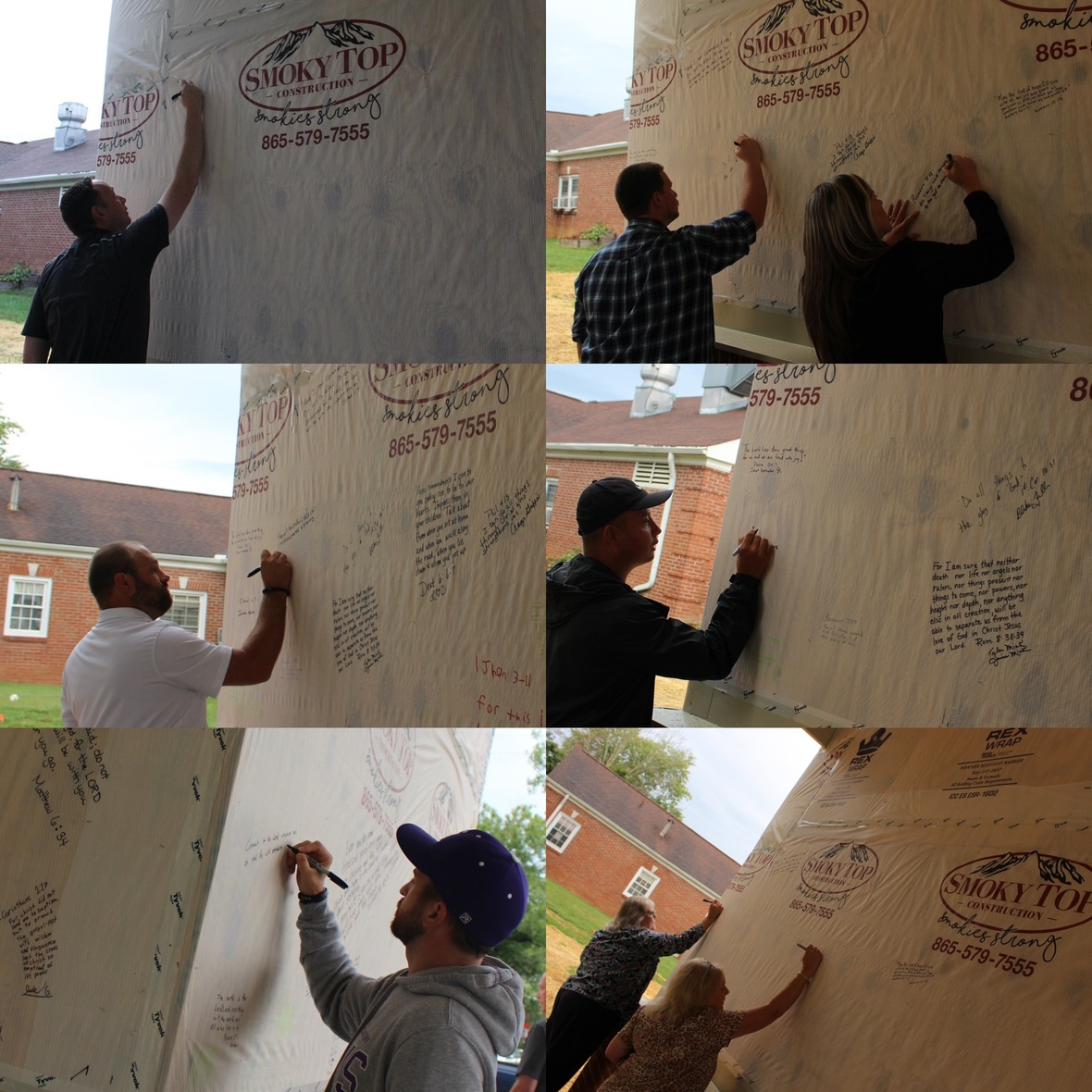table div table+table+table+table+table+table+table+table+table+table div table{width:100%;padding:0}table div table+table+table+table+table+table+table+table+table+table div table img{width:96.23%;padding:0;float:none}table div table+table+table+table+table+table+table+table+table+table div table td{width:100%;padding:0 1.88% 18px}/* styles */# Love Where We Live T-Shirt

All TKA students, faculty, and staff will receive a Love Where We Live t-shirt free of charge! These shirts are what will be worn on our Love Where We Live service project days. All that is needed is each person’s shirt size. Please fill out one form per TKA Student and return it to your homeroom/1st period teacher by Friday, September 24.

Any family or friends of TKA that would like to order a t-shirt, may do so for \$10 per shirt. Click here to download the family and friends of TKA order form.

 table div table+table+table+table+table+table+table+table+table+table+table+table div table{width:100%;padding:0}table div table+table+table+table+table+table+table+table+table+table+table+table div table img{width:96.23%;padding:0;float:none}table div table+table+table+table+table+table+table+table+table+table+table+table div table td{width:100%;padding:0 1.88% 18px}/* styles */# Hawaiian Dress Up Day

This Friday, September 24, is Hawaiian dress up day! Students can dress in their best Hawaiian attire.
Remember, dress out day expectations are as follows:
▪ Dress code length (shorts/skirts, etc.) is expected
▪ Dress code footwear is expected
▪ Jeans are allowed (no rips, tears, etc.)
▪ No inappropriate, vulgar, or any print that goes against the mission and vision of TKA is allowed
▪ Hats are allowed but are to be removed in the classroom if asked to allow all students visual access to presented materials, etc.

 /* styles */
 table div table+table+table+table+table+table+table+table+table+table+table+table+table+table+table div table{width:100%;padding:0}table div table+table+table+table+table+table+table+table+table+table+table+table+table+table+table div table img{width:96.23%;padding:0;float:none}table div table+table+table+table+table+table+table+table+table+table+table+table+table+table+table div table td{width:100%;padding:0 1.88% 18px}/* styles */# Preview Night

Do you have any friends or neighbors who would benefit from hearing more about The King's Academy family? If so, invite them to join us on Preview Night next Friday, October 1. TKA will provide a BBQ meal and complimentary tickets to the game for all prospective families who attend. We want to share with families why TKA is a great choice for Christ-centered K3-12th grade education!

 table div table+table+table+table+table+table+table+table+table+table+table+table+table+table+table+table+table div table{width:100%;padding:0}table div table+table+table+table+table+table+table+table+table+table+table+table+table+table+table+table+table div table img{width:96.23%;padding:0;float:none}table div table+table+table+table+table+table+table+table+table+table+table+table+table+table+table+table+table div table td{width:100%;padding:0 1.88% 18px}/* styles */# Breast Cancer Awareness

In honor of Breast Cancer Awareness Month, we will be having a Pink Out Day on Friday, October 1, throughout the school day and at the football game. During halftime, we will recognize breast cancer survivors with their families on the football field, as well as honor those families who have lost a loved one due to breast cancer. If you would like to honor someone during halftime, please fill out this form.

On Saturday, October 2, at 10:00 a.m. a breast cancer walk will take place on the campus of TKA. This walk is to honor those who have fought and are continuing to fight breast cancer. We will also be collecting money for someone currently undergoing breast cancer treatments. Please join us as we celebrate with breast cancer survivors, their families and their support teams. We will also honor loved ones lost to breast cancer. There will be some food, music, and pictures will be taken. Masks are encouraged but not required for this event. To sign up for this event, click here.

If you have any questions about either event, please call Jennifer Ayers at (865) 202-8989.

 table div table+table+table+table+table+table+table+table+table+table+table+table+table+table+table+table+table+table+table div table{width:100%;padding:0}table div table+table+table+table+table+table+table+table+table+table+table+table+table+table+table+table+table+table+table div table img{width:96.23%;padding:0;float:none}table div table+table+table+table+table+table+table+table+table+table+table+table+table+table+table+table+table+table+table div table td{width:100%;padding:0 1.88% 18px}/* styles */# Elementary R.O.A.R. t-shirt

We are excited to offer our 2nd annual ROAR Elementary t-shirt. These shirts may be worn on Fridays with the approved dress code.

The front of the shirt will include our school lion logo with our elementary theme R.O.A.R. (Radical Love for Jesus and Others; Opportunities to serve; Show Christ-like Attitude; Speak with Respectful Language). On the back, we will have the year 2021 along with the scripture Galatians 5:22-23. Inside the year, parents can sponsor to have their child’s signature printed.

The annual shirt will provide students a keepsake with each year they attended elementary school along with the opportunity to see the growth of their signature over the years.

The ROAR fundraiser helps us continue to provide resources and support for our teachers and staff in the elementary.

 table div table+table+table+table+table+table+table+table+table+table+table+table+table+table+table+table+table+table+table+table+table div table{width:100%;padding:0}table div table+table+table+table+table+table+table+table+table+table+table+table+table+table+table+table+table+table+table+table+table div table img{width:96.23%;padding:0;float:none}table div table+table+table+table+table+table+table+table+table+table+table+table+table+table+table+table+table+table+table+table+table div table td{width:100%;padding:0 1.88% 18px}/* styles */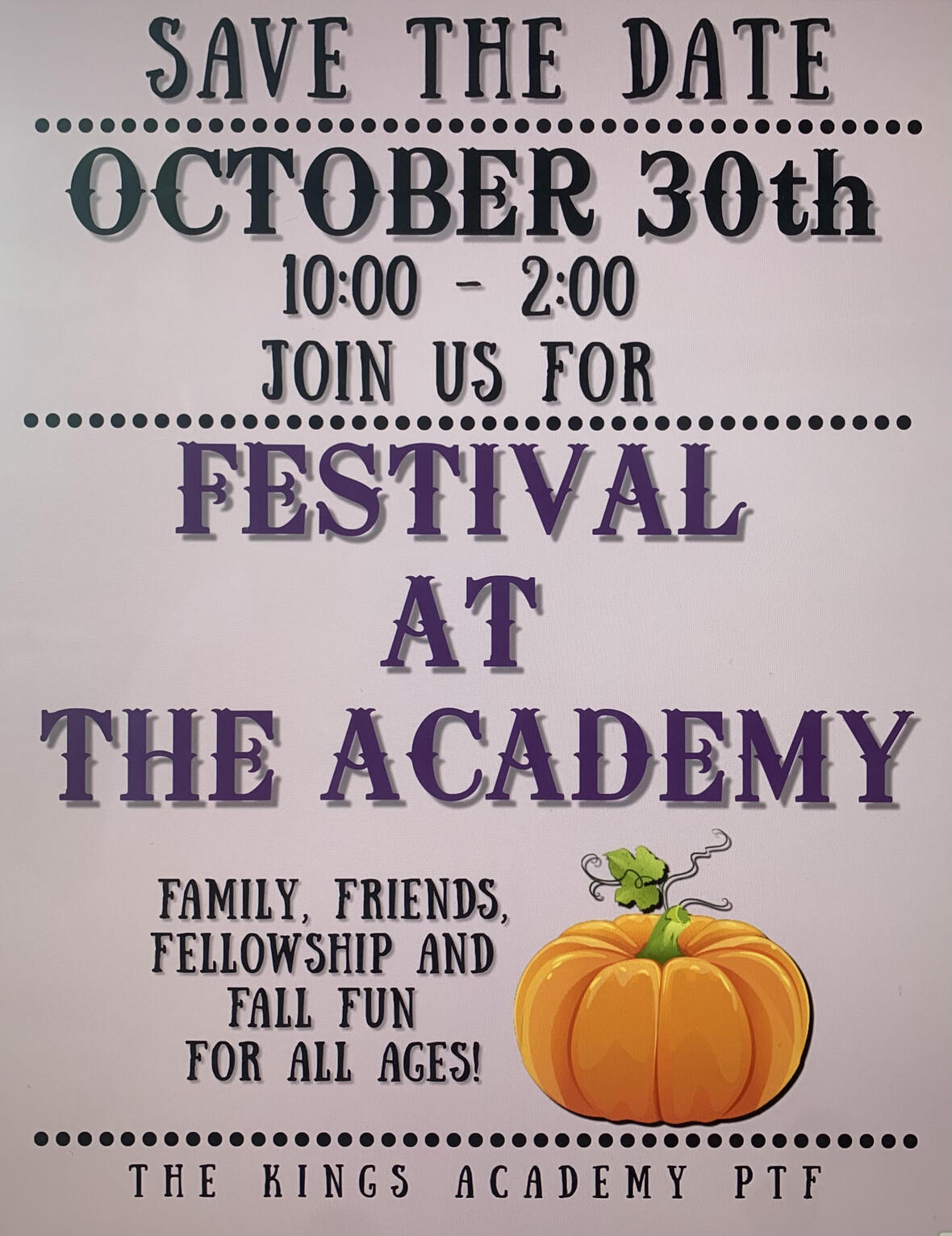Mark your calendars and save the date! The Festival at the Academy is taking place on Saturday, October 30 from 10 a.m. to 2 p.m.

The committee is currently taking donations for the silent auction baskets. If you or your company would like to donate a tax deductible basket, reach out to one of the contacts listed below!

## Contacts for Festival at The Academy:

The King’s Academy (865) 573-8321 ext.5
Kara Pritchard (865) 924-4333
Cherish McLin (865) 604-8303

 table div table+table+table+table+table+table+table+table+table+table+table+table+table+table+table+table+table+table+table+table+table+table+table div table{width:100%;padding:0}table div table+table+table+table+table+table+table+table+table+table+table+table+table+table+table+table+table+table+table+table+table+table+table div table img{width:96.23%;padding:0;float:none}table div table+table+table+table+table+table+table+table+table+table+table+table+table+table+table+table+table+table+table+table+table+table+table div table td{width:100%;padding:0 1.88% 18px}/* styles */table div table+table+table+table+table+table+table+table+table+table+table+table+table+table+table+table+table+table+table+table+table+table+table+table+table div table{width:100%;padding:0}table div table+table+table+table+table+table+table+table+table+table+table+table+table+table+table+table+table+table+table+table+table+table+table+table+table div table img{width:96.23%;padding:0;float:none}table div table+table+table+table+table+table+table+table+table+table+table+table+table+table+table+table+table+table+table+table+table+table+table+table+table div table td{width:100%;padding:0 1.88% 18px}/* styles */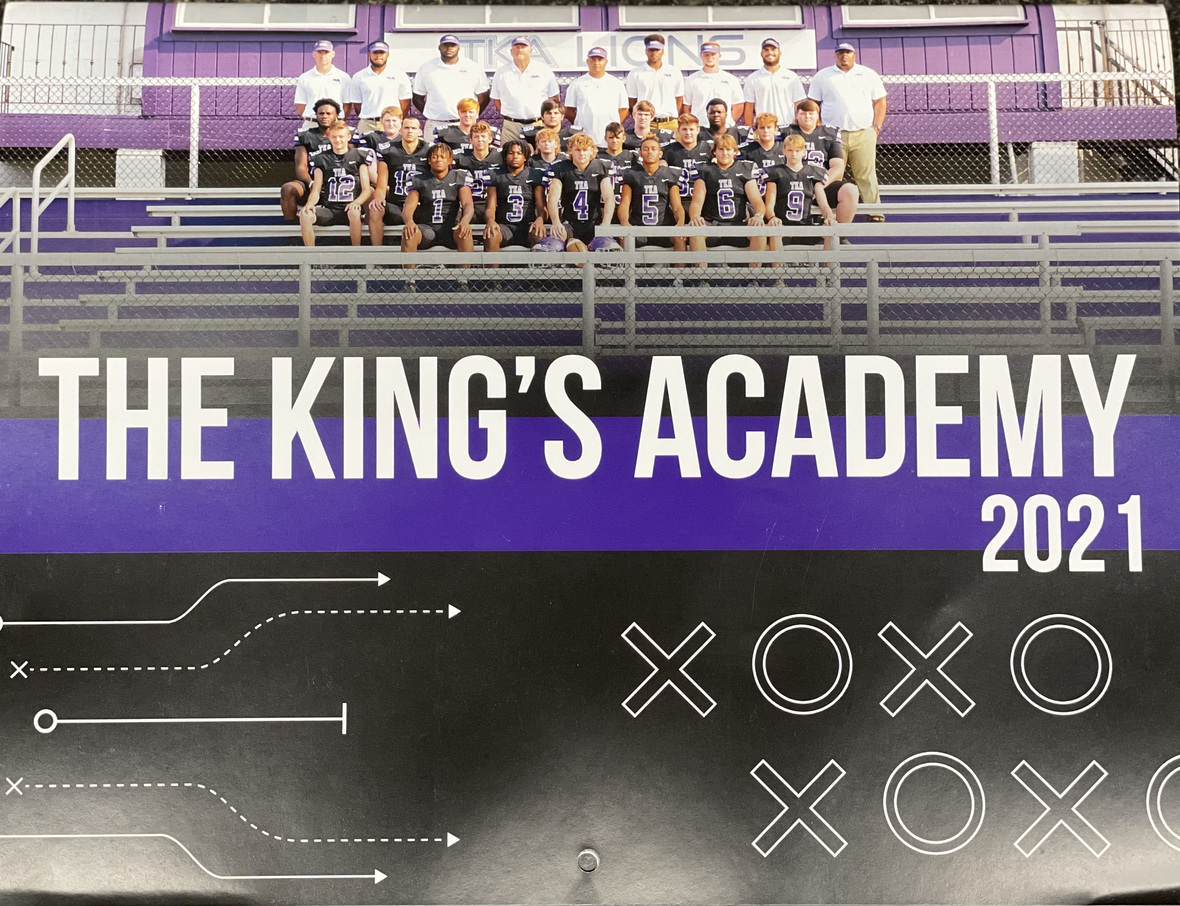# 2021-2022 Football Calendar

This year's football calendars are for sale for \$10! Stop by the school office to pick up your copy today!

 table div table+table+table+table+table+table+table+table+table+table+table+table+table+table+table+table+table+table+table+table+table+table+table+table+table+table+table div table{width:100%;padding:0}table div table+table+table+table+table+table+table+table+table+table+table+table+table+table+table+table+table+table+table+table+table+table+table+table+table+table+table div table img{width:96.23%;padding:0;float:none}table div table+table+table+table+table+table+table+table+table+table+table+table+table+table+table+table+table+table+table+table+table+table+table+table+table+table+table div table td{width:100%;padding:0 1.88% 18px}/* styles */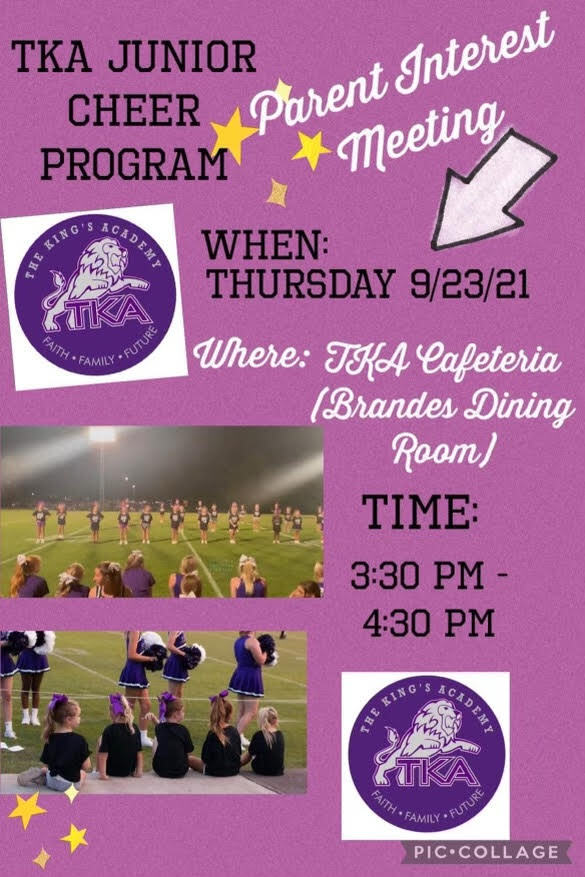# TKA Junior Cheer Parent Meeting

 table div table+table+table+table+table+table+table+table+table+table+table+table+table+table+table+table+table+table+table+table+table+table+table+table+table+table+table+table+table div table{width:100%;padding:0}table div table+table+table+table+table+table+table+table+table+table+table+table+table+table+table+table+table+table+table+table+table+table+table+table+table+table+table+table+table div table img{width:96.23%;padding:0;float:none}table div table+table+table+table+table+table+table+table+table+table+table+table+table+table+table+table+table+table+table+table+table+table+table+table+table+table+table+table+table div table td{width:100%;padding:0 1.88% 18px}/* styles */Is your child interested in playing basketball for TKA's Jr. League teams this year? Please complete this brief 'TKA Jr. League Basketball Interest Form' that will assist us in determining interest and developing preliminary rosters. Once completed, our Jr. League leadership team will evaluate the responses and reach out to you with additional information.

Jr. League is a competitive elementary basketball league which typically consists of 1-2 practices per week. Historically there are 10 regular season games which take place on Saturdays in gyms throughout Sevier County (TKA included). The season typically begins in early November and concludes around mid-February.

We look forward to hearing from you!

Marshall Stanger
TKA Junior League Director
tkahoops@gmail.com
865.566.7928

 table div table+table+table+table+table+table+table+table+table+table+table+table+table+table+table+table+table+table+table+table+table+table+table+table+table+table+table+table+table+table+table div table{width:100%;padding:0}table div table+table+table+table+table+table+table+table+table+table+table+table+table+table+table+table+table+table+table+table+table+table+table+table+table+table+table+table+table+table+table div table img{width:96.23%;padding:0;float:none}table div table+table+table+table+table+table+table+table+table+table+table+table+table+table+table+table+table+table+table+table+table+table+table+table+table+table+table+table+table+table+table div table td{width:100%;padding:0 1.88% 18px}/* styles */# Thank you!

A big thank you from the library for all the support you gave through our Scholastic Book Fair. We sold over 500 books and raised more than \$1000 for our library!

 table div table+table+table+table+table+table+table+table+table+table+table+table+table+table+table+table+table+table+table+table+table+table+table+table+table+table+table+table+table+table+table+table+table div table{width:100%;padding:0}table div table+table+table+table+table+table+table+table+table+table+table+table+table+table+table+table+table+table+table+table+table+table+table+table+table+table+table+table+table+table+table+table+table div table img{width:96.23%;padding:0;float:none}table div table+table+table+table+table+table+table+table+table+table+table+table+table+table+table+table+table+table+table+table+table+table+table+table+table+table+table+table+table+table+table+table+table div table td{width:100%;padding:0 1.88% 18px}/* styles */table.module-33{width:99.81%;padding:0}table div table+table+table+table+table+table+table+table+table+table+table+table+table+table+table+table+table+table+table+table+table+table+table+table+table+table+table+table+table+table+table+table+table+table div table{width:99.81%;float:none;margin-left:auto;margin-right:auto;padding:0}table div table+table+table+table+table+table+table+table+table+table+table+table+table+table+table+table+table+table+table+table+table+table+table+table+table+table+table+table+table+table+table+table+table+table div table a{border:0 none;text-decoration:none}table div table+table+table+table+table+table+table+table+table+table+table+table+table+table+table+table+table+table+table+table+table+table+table+table+table+table+table+table+table+table+table+table+table+table div table img{width:100%!important;border:0 none;text-decoration:none}table div table+table+table+table+table+table+table+table+table+table+table+table+table+table+table+table+table+table+table+table+table+table+table+table+table+table+table+table+table+table+table+table+table+table div table td{width:100%;padding:0}/* styles */
 table div table+table+table+table+table+table+table+table+table+table+table+table+table+table+table+table+table+table+table+table+table+table+table+table+table+table+table+table+table+table+table+table+table+table+table div table{width:100%;padding:0}table div table+table+table+table+table+table+table+table+table+table+table+table+table+table+table+table+table+table+table+table+table+table+table+table+table+table+table+table+table+table+table+table+table+table+table div table img{width:96.23%;padding:0;float:none}table div table+table+table+table+table+table+table+table+table+table+table+table+table+table+table+table+table+table+table+table+table+table+table+table+table+table+table+table+table+table+table+table+table+table+table div table td{width:100%;padding:0 1.88% 18px}/* styles */# Covid-19 Procedures

While we are excited to offer in-person education, we must continue to maintain a safe learning environment for all students, faculty/staff, and additional stakeholders.

To do so, we will be working closely with the Sevier County Health Department in regards to Covid-19 protocols (https://seviercountycovidhelp.com/).

 table div table+table+table+table+table+table+table+table+table+table+table+table+table+table+table+table+table+table+table+table+table+table+table+table+table+table+table+table+table+table+table+table+table+table+table+table+table div table{width:100%;padding:0}table div table+table+table+table+table+table+table+table+table+table+table+table+table+table+table+table+table+table+table+table+table+table+table+table+table+table+table+table+table+table+table+table+table+table+table+table+table div table img{width:96.23%;padding:0;float:none}table div table+table+table+table+table+table+table+table+table+table+table+table+table+table+table+table+table+table+table+table+table+table+table+table+table+table+table+table+table+table+table+table+table+table+table+table+table div table td{width:100%;padding:0 1.88% 18px}/* styles */table div table+table+table+table+table+table+table+table+table+table+table+table+table+table+table+table+table+table+table+table+table+table+table+table+table+table+table+table+table+table+table+table+table+table+table+table+table+table+table div table{width:100%;padding:0}table div table+table+table+table+table+table+table+table+table+table+table+table+table+table+table+table+table+table+table+table+table+table+table+table+table+table+table+table+table+table+table+table+table+table+table+table+table+table+table div table img{width:96.23%;padding:0;float:none}table div table+table+table+table+table+table+table+table+table+table+table+table+table+table+table+table+table+table+table+table+table+table+table+table+table+table+table+table+table+table+table+table+table+table+table+table+table+table+table div table td{width:100%;padding:0 1.88% 18px}/* styles */